# 2 Answers for circuit analysis question, both seem right.

#### John_iqbal786

Joined Nov 3, 2019
7
The question is asking what the resistance of the first resistor is (which has been labelled as 0 ohms on the diagram). I am told 20v drops across the first resistor and i am given the value of the other resistor. Now both of the methods I am sure I've done correctly but I'm getting different answers.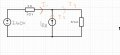In this first method, I simply calculated the current through the 57.6 ohm resistor, which was 220/57.6. To find I1, I would do I3-I2. Which is (220/57.6)-10 =6.18A. This is the current through the first resistor. Therefore to find its resistance I would do V/I=R.
So it is 20/6.18 = 3.24 Ohms

I was happy with my answer until I decided to try another method, which was to transform the current source into a voltage source and analyse it that way.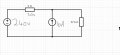To transform to voltage source. I did 10 * 57.6 = 576V. As a current source in parallel with a resistor can be transformed into a voltage source in series with the resistor.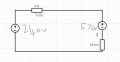Here I then combined the voltage sources which would be 576-240 = 336V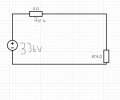From here I then worked out the current through the 57.6 ohm resistor (which would also be the current through the first resistor). Since 20v is across the first resistor, then 316v would be across the 57.6 ohm resistor. Therefore I = 316/57.6 = 5.4861
Using this value for current, I then used ohms law to work out the resistance of the first resistor. Since R = V/I, I did 20/5.4861 = 3.65 Ohms

Here's my dilemma, both methods seem to be done correctly and should both work to give the same answer, but the first method gives me 3.24 Ohms and the second gives me 3.65 Ohms. I am so confused right now, which method is the correct one and which answer is correct? What did I do wrong.

#### WBahn

Joined Mar 31, 2012
27,876
The last time I checked, (220/57.6)-10 was not equal to 6.18.

#### John_iqbal786

Joined Nov 3, 2019
7
The last time I checked, (220/57.6)-10 was not equal to 6.18.
Yes I realise it is -6.18A, but since i used it to work out the value of the resistor via R=V/I, then I dont think it makes a difference. The current flowing through the first resistor is still 6.18A, the direction of the current wont change the value of the resistance.

#### WBahn

Joined Mar 31, 2012
27,876
Yes I realise it is -6.18A, but since i used it to work out the value of the resistor via R=V/I, then I dont think it makes a difference.
It only makes the difference between a right answer and a wrong answer.

You are making a bad assumption in the set up of your analysis and the math is SCREAMING out to you that you have made a bad assumption, and you are choosing to ignore it and barge ahead.

If you are going to ignore what the math is telling you, then why bother doing it at all?

•djsfantasi

#### John_iqbal786

Joined Nov 3, 2019
7
It only makes the difference between a right answer and a wrong answer.

You are making a bad assumption in the set up of your analysis and the math is SCREAMING out to you that you have made a bad assumption, and you are choosing to ignore it and barge ahead.

If you are going to ignore what the math is telling you, then why bother doing it at all?
I am not ignoring what the math is telling me? In the set up of my analysis, I assumed the current I1 to be moving towards the right, after completing the analysis, I have found the current is -6.18A which is telling me it is 6.18A in the opposite direction (to the left).

However, since I already have the voltage of my resistor, my goal is to find the value of the current travelling through that same resistor which will then let me find resistance. I have found out the current that is flowing through that resistor is 6.18A which I now know is flowing towards the left and not the right (thanks to the negative sign), but regardless the current flowing through that resistor is still 6.18A and so will not affect the value for my resistance? You are saying I am barging through the question, but if I had taken the current of I1 to be in the opposite direction from the beginning my result for I1 would then be +6.18A and Still my answer for resistance will not change?

#### WBahn

Joined Mar 31, 2012
27,876
I am not ignoring what the math is telling me? In the set up of my analysis, I assumed the current I1 to be moving towards the right, after completing the analysis, I have found the current is -6.18A which is telling me it is 6.18A in the opposite direction (to the left).

However, since I already have the voltage of my resistor, my goal is to find the value of the current travelling through that same resistor which will then let me find resistance. I have found out the current that is flowing through that resistor is 6.18A which I now know is flowing towards the left and not the right (thanks to the negative sign), but regardless the current flowing through that resistor is still 6.18A and so will not affect the value for my resistance? You are saying I am barging through the question, but if I had taken the current of I1 to be in the opposite direction from the beginning my result for I1 would then be +6.18A and Still my answer for resistance will not change?
You started off assuming that the current was flowing through the resistor left-to-right and BASED ON THAT ASSUMPTION you determined that the voltage on the right side of that resistor was 220 V.

But the math told you that the assumption that the current was flowing through the resistor left-to-right was a BAD assumption, yet you are content to keep assuming that the voltage on the right side of that resistor is 220 V, which is only possible if the current in it is flowing left-to-right, even though the math told you that that assumption was BAD. So, yes, I maintain that you are ignoring what the math is telling you and just barging on through the question.

•djsfantasi

#### John_iqbal786

Joined Nov 3, 2019
7
You started off assuming that the current was flowing through the resistor left-to-right and BASED ON THAT ASSUMPTION you determined that the voltage on the right side of that resistor was 220 V.

But the math told you that the assumption that the current was flowing through the resistor left-to-right was a BAD assumption, yet you are content to keep assuming that the voltage on the right side of that resistor is 220 V, which is only possible if the current in it is flowing left-to-right, even though the math told you that that assumption was BAD. So, yes, I maintain that you are ignoring what the math is telling you and just barging on through the question.
As I said before the question stated that the Voltage across the first resistor is 20V. The voltage source is 240V, therefore the voltage across the 57.6ohm resistor is indeed 220V?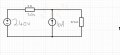I have redrawn it so that the current is flowing in the correct directions. Now the voltage across the first resistor is still 20V (As I was told this in the questions) and so the voltage across the 57.6 ohm resistor Still is 220V. The value for I3 will still be 220/57.6. And now I can see that I2 = I1 +I3, so I1= I2-I3 = 10 - (220/57.6) = (+) 6.18 A. and so I still have the same current through the first resistor and my answer for resistance is still unchanged.

You are saying that I decided the 57.6 ohm resistor had a voltage of 220V based on my assumption that current was flowing from left to right. My reasoning for assigning 220V to the 57.6ohm resistor was that since the first resistor has a voltage of 20V then the 57.6 ohm resistor must have a voltage of 220V since the source is 240V.
Now that the current is flowing from right to left, the voltage of the first resistor is still 20V so why would the voltage of the 57.6ohm resistor not still be 220V, if there is something I am missing here please let me know as I am confused.

#### WBahn

Joined Mar 31, 2012
27,876
If current is flowing into a resistor from side A and out through side B, which side of the resistor has a higher voltage? Side A or side B?

•djsfantasi

#### John_iqbal786

Joined Nov 3, 2019
7
If current is flowing into a resistor from side A and out through side B, which side of the resistor has a higher voltage? Side A or side B?
Side A will have the higher voltage. Which I Guess is shown in my second method when I transformed the current source into the voltage source. Looking back after I combined them the 336V voltage source I ended up with should have its polarity flipped the other way but the answer for the value of the resistor at that stage would still be the same.

For my first method I am unsure what to do with the extra information that the right side of the resistor has a higher voltage than the left side. Someone has mentioned that I can write the voltage of the first resistor as (-)20V and the voltage of the resistor on the right as (+)260V while the voltage source is still the same (+)240V. This would still ensure that the voltage on the right side of the reistor is larger than that of the left side. But, I have never used negative voltages when working with circuits analysis. Is this what you have been trying to hint at? If I did mark the 20V as -20V and the 57.6ohm resistor as 260V then the answer for resistance of the first resistor is indeed the same as my second method.

#### Dodgydave

Joined Jun 22, 2012
10,591
How can you have 20V drop across a Short Circuit??

#### John_iqbal786

Joined Nov 3, 2019
7
How can you have 20V drop across a Short Circuit??
It is not a short circuit. The question is asking for me to work out the resistance of the first resistor. It is just labelled as s 0 ohms as it wasn't possible to label it as R. The resistance of that resistor is not 0, it is unknown and it is what I am trying to figure out. (they tell you the resistors voltage is 20v)

#### WBahn

Joined Mar 31, 2012
27,876
Side A will have the higher voltage. Which I Guess is shown in my second method when I transformed the current source into the voltage source. Looking back after I combined them the 336V voltage source I ended up with should have its polarity flipped the other way but the answer for the value of the resistor at that stage would still be the same.

For my first method I am unsure what to do with the extra information that the right side of the resistor has a higher voltage than the left side. Someone has mentioned that I can write the voltage of the first resistor as (-)20V and the voltage of the resistor on the right as (+)260V while the voltage source is still the same (+)240V. This would still ensure that the voltage on the right side of the reistor is larger than that of the left side. But, I have never used negative voltages when working with circuits analysis. Is this what you have been trying to hint at? If I did mark the 20V as -20V and the 57.6ohm resistor as 260V then the answer for resistance of the first resistor is indeed the same as my second method.
The key thing that will help deal with these kinds of issues is to clearly annotate your diagrams with all voltages and currents that you use. That way if you do end up with a negative value its meaning is unambiguous. The bottom line on your first analysis is that you didn't end up with R = 3.24 Ω. You only got that because you threw out a minus sign because you saw it as inconvenient. Had you not done that you would have gotten R = -3.24 Ω which would have screamed out that it could not be the correct answer and you would have then (hopefully) gone looking for what assumption you were making that must be incorrect.

For this particular problem, the critical observation is that when they say that the voltage drop across a resistor is 20 V that they are not giving enough information to determine the polarity of the voltage drop, only its magnitude. Thus you have to allow for both possibilities. The real point to learn from that is that if just being told that the voltage drop across a resistor is 20 V is not complete enough for you to know exactly what they mean, then if you talk about the voltage drop across something being some value is also not complete enough for your audience (be it a customer or a grader) to know exactly what you mean, either.

Consider the following annotated diagram for the problem: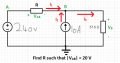Now we have the following equations that describe the circuit:

Va = 240 V
I2 = 10 A
I3 = I1 + I2
I1 = Vab/R
I3 = Vb/(57.6 Ω)
Vab = Va - Vb

So now we can start eliminating variables until we get R in terms of Vab.

I3 = I1 + I2
Vb/(57.6 Ω) = Vab/R + (10 A)
(Va - Vab)/(57.6 Ω) = Vab/R + (10 A)
R = Vab / [(Va - Vab)/(57.6 Ω) - (10 A)]

For |Vab| = 20 V we have two possibilities.
Vab = +20 V => R = -3.24 Ω
Vab = -20 V => R = +3.65 Ω

Of these two, only the second is possible, so we conclude that Vab = -20 V, making Vb= 260 V.

Also, notice how the units were tracked properly throughout each and every step of the work. One of the things this allows is for quick units sanity checks at each step. Looking at just the final equation, we have

R = Vab / [(Va - Vab)/(57.6 Ω) - (10 A)]

Voltage minus a voltage is a voltage.

R = Vab / [(Va - Vab)/(57.6 Ω) - (10 A)]

Voltage over a resistance is a current.

R = Vab / [(Va - Vab)/(57.6 Ω) - (10 A)]

Current minus a current is a current.

R = Vab / [(Va - Vab)/(57.6 Ω) - (10 A)]

Voltage over a current is a resistance, which is what we need to match the left-hand side.

Last edited:

#### John_iqbal786

Joined Nov 3, 2019
7
The key thing that will help deal with these kinds of issues is to clearly annotate your diagrams with all voltages and currents that you use. That way if you do end up with a negative value its meaning is unambiguous. The bottom line on your first analysis is that you didn't end up with R = 3.24 Ω. You only got that because you threw out a minus sign because you saw it as inconvenient. Had you not done that you would have gotten R = -3.24 Ω which would have screamed out that it could not be the correct answer and you would have then (hopefully) gone looking for what assumption you were making that must be incorrect.

For this particular problem, the critical observation is that when they say that the voltage drop across a resistor is 20 V that they are not giving enough information to determine the polarity of the voltage drop, only its magnitude. Thus you have to allow for both possibilities. The real point to learn from that is that if just being told that the voltage drop across a resistor is 20 V is not complete enough for you to know exactly what they mean, then if you talk about the voltage drop across something being some value is also not complete enough for your audience (be it a customer or a grader) to know exactly what you mean, either.

Consider the following annotated diagram for the problem:
View attachment 190639

Now we have the following equations that describe the circuit:

Va = 240 V
I2 = 10 A
I3 = I1 + I2
I1 = Vab/R
I3 = Vb/(57.6 Ω)
Vab = Va - Vb

So now we can start eliminating variables until we get R in terms of Vab.

I3 = I1 + I2
Vb/(57.6 Ω) = Vab/R + (10 A)
(Va - Vab)/(57.6 Ω) = Vab/R + (10 A)
R = Vab / [(Va - Vab)/(57.6 Ω) - (10 A)]

For |Vab| = 20 V we have two possibilities.
Vab = +20 V => R = -3.24 Ω
Vab = -20 V => R = +3.65 Ω

Of these two, only the second is possible, so we conclude that Vab = -20 V, making Vb= 260 V.

Also, notice how the units were tracked properly throughout each and every step of the work. One of the things this allows is for quick units sanity checks at each step. Looking at just the final equation, we have

R = Vab / [(Va - Vab)/(57.6 Ω) - (10 A)]

Voltage minus a voltage is a voltage.

R = Vab / [(Va - Vab)/(57.6 Ω) - (10 A)]

Voltage over a resistance is a current.

R = Vab / [(Va - Vab)/(57.6 Ω) - (10 A)]

Current minus a current is a current.

R = Vab / [(Va - Vab)/(57.6 Ω) - (10 A)]

Voltage over a current is a resistance, which is what we need to match the left-hand side.
Thank you for the detailed response. I never realised the current would be different based on the whether the voltage is positive or negative in the resistor. This is the first question I've come across where it has made a difference. I'll always be redoing my diagrams and analysis once I find the correct direction for current.
Thank you again

#### RBR1317

Joined Nov 13, 2010
706
I'll always be redoing my diagrams and analysis once I find the correct direction for current.
Problems like this no doubt help to develop intuition about the behavior of voltages and currents; however, there are formalized methods that avoid the need for such intuition. In nodal analysis, the currents at a node must add up to equal zero, and the currents are expressed in terms of the node voltages. Each term of the node equation takes the form: (Node voltage – Adjacent node voltage)/(Resistance to the adjacent node). With this convention, defined currents (current source) leaving a node are positive; defined currents entering a node are negative. That's all there is to nodal analysis.

In this problem there is one node with unknown voltage, but is known to be 240±20 V, so the node equation would be ((240±20)-240)/R -10A +(240±20)/57.6Ω = 0. There will be two equations to solve (one for +20 & one for -20). There will be two solutions: one with R positive, one with R negative.

#### MrAl

Joined Jun 17, 2014
9,633
Hello,

Remove the current source. What are we left with?
240-vR=220
220/57.6 = 275/72 amps = 3.819444444444445 amps approximate = 3.82 amps more approximate.

Now with the current source back in the circuit that means that 6.18 amps must be flowing from right to left in R.
Since one of the assumptions was that the voltage drop in R was positive left and negative right, that makes the resistor R have a negative resistance because a positive current is flowing into a negative voltage terminal.
Now if we change the assumption such that the voltage drop is negative on left and positive on right then the voltage across the 57.6 Ohm resistor is 260v and then we should get a regular resistor value.

Check this out and see what you think. I wont give any results just yet.